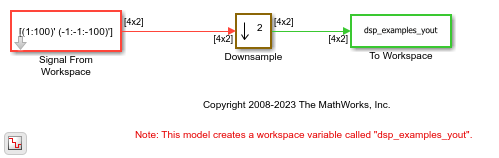# Downsample a Signal

Downsample a signal by a factor of 2 using the Downsample block.

### Open the System and Run the ModelThe Signal From Workspace block generates a two-channel signal with a frame size of 4. The two Probe (Simulink) blocks are specified to show the sample time of the signal before and after the downsampling operation.

Run the model. The sample time of the signal before the downsampling operation is half the sample time of the signal after the operation. You can see this from the `Ts` parameter visible on the two Probe blocks.

### One-Frame Latency

The Input processing parameter in the Downsample block is set to `Columns as channels (frame based)` and the input frame size (number of rows in the input), Mi, is greater than 1. Hence, the latency of the signal is one frame. The Initial conditions parameter is set to `[11 -11;12 -12;13 -13;14 -14]`. In all cases of one-frame latency, the Mi rows of the initial condition matrix appear in sequence as the first four output rows. Input sample D+1 (i.e, row D+1 of the input matrix) appears in the output as sample Mi+1, followed by input sample D+1+K, input sample D+1+2K, and so on.

• Mi - Number of input rows. In this example, Mi equals 4.

• D - Sample offset parameter. In this example, D equals 1.

• K - Downsample factor. In this example, K equals 2.

The Initial conditions value can be an Mi-by- N matrix containing one value for each channel, or a scalar to be repeated across all elements of the Mi-by- N matrix.

Here is the downsampled output signal written to the `dsp_examples_yout` variable in the base workspace.

```dsp_examples_yout = 11 -11 12 -12 13 -13 14 -14 2 -2 4 -4 6 -6 8 -8 10 -10 12 -12 14 -14 16 -16 18 -18 20 -20 22 -22 24 -24 26 -26 28 -28 30 -30 32 -32 34 -34 36 -36 38 -38 40 -40 42 -42 44 -44 46 -46 48 -48 50 -50 52 -52 54 -54 56 -56 58 -58 60 -60 62 -62 64 -64 66 -66 68 -68 70 -70 72 -72 74 -74 76 -76 78 -78 80 -80 82 -82 84 -84 86 -86 88 -88 90 -90 92 -92 94 -94 96 -96 98 -98 100 -100 0 0 0 0 0 0 0 0 0 0 0 0 0 0 0 0 0 0 0 0 ```